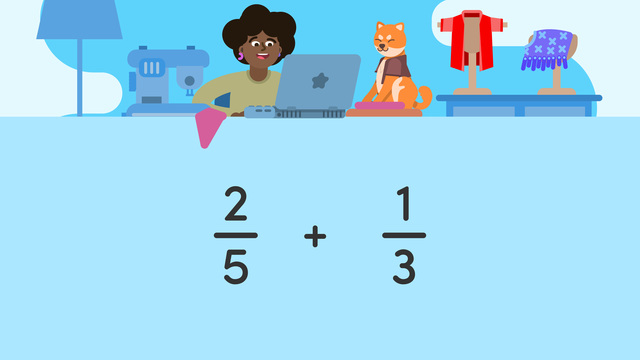# Adding Fractions with Unlike Denominators

Content Adding Fractions with Unlike DenominatorsRating

Ø 4.7 / 3 ratings
The authorsTeam Digital
CCSS.MATH.CONTENT.5.NF.A.1

## Adding Fractions with Unlike Denominators

Looking for a comprehensive and engaging way to help your 5th grader master the concept of adding fractions with unlike denominators? Look no further than our tutorial video on adding fractions with unlike denominators by Sofatutor!

Designed specifically for 5th graders, this video is the perfect tool to help your child understand the fundamentals of adding fractions with different denominators. With clear explanations, helpful examples, and interactive practice problems, our video is sure to keep your child engaged and motivated as they learn.

Whether your child is struggling with this concept or simply looking for a new way to learn, our tutorial video by Sofatutor is the perfect solution. So why wait? Start your child's journey to math mastery today with our adding fractions with unlike denominators video! With our engaging and informative content, your child will be well on their way to understanding this important mathematical concept in no time.

## Adding Fractions with Unlike Denominators exercise

Would you like to apply the knowledge you’ve learned? You can review and practice it with the tasks for the video Adding Fractions with Unlike Denominators .
• ### Define the terms.

Hints

A denominator is part of a fraction.

Look for common words on left and right sides to help you make matches.

Think of the word least as small, and the word greatest as large.

Solution
• The Least Common Multiple, or LCM is smallest multiple that two numbers have in common.
• The Least Common Denominator, or LCD is the smallest number that can be used to make equal denominators in fractions.
• The Greatest Common Factor or GCF is the largest number that can be divided evenly into 2 or more numbers.
• The Denominator is the number below the fraction line.
• ### Sort the steps.

Hints

The numerator is the number above the fraction line, and the denominator is the number below.

Before adding fractions with unlike denominators, you need to create a common denominator using multiplication or division.
Example: $\frac{1}{2}$ and $\frac{1}{4}$
$\frac{1}{2}$ x $\frac{2}{2}$ = $\frac{2}{4}$
$\frac{2}{4}$ and $\frac{1}{4}$ have common denominators and can now be added together.

Solution

The steps to add fractions with unlike denominators are:
1) Rename fractions with unlike denominators as equivalent fractions with the same denominator ($\frac{1}{12}$ + $\frac{1}{4}$ becomes $\frac{1}{12}$ + $\frac{3}{12}$)
2) Bring the common denominator over to the solution side of the equation ($\frac{1}{12}$ + $\frac{3}{12}$ = $\frac{}{12}$)
3) Add the numerators ($\frac{1}{12}$ + $\frac{3}{12}$ = $\frac{4}{12}$)
4) Simplify the fraction if necessary ($\frac{4}{12}$ becomes $\frac{1}{3}$)

• ### Add fractions with unlike denominators.

Hints

Sometimes the least common denominator is already the denominator of one of the fractions in the equation.

Starting in step one, the Least Common Denominator (15) is given. What do you multiply each fraction by to get 15 in the denominator?

You have the solution for $\frac{3}{15}$ + $\frac{2}{15}$ = ? from step 3. Now divide both the numerator and denominator of this fraction by 5 to simplify.

Solution

STEP ONE
$\frac{1}{5}$ x $\frac{3}{3}$ = $\frac{3}{15}$
$\frac{2}{15}$ x $\frac{1}{1}$ = $\frac{2}{15}$
STEP TWO
$\frac{3}{15}$ + $\frac{2}{15}$ = $\frac{}{15}$
STEP THREE
$\frac{3}{15}$ + $\frac{2}{15}$ = $\frac{5}{15}$
STEP FOUR
$\frac{5}{15}$ ÷ $\frac{5}{5}$ = $\frac{1}{3}$

• ### Solve the equations.

Hints

In order to add fractions with unlike denominators, first create equivalent fractions with the same denominator using multiplication or division.
Example:
$\frac{1}{5}$ + $\frac{2}{15}$
$\frac{1}{5}$ x $\frac{3}{3}$ = $\frac{3}{15}$
$\frac{2}{15}$ x $\frac{1}{1}$ = $\frac{2}{15}$
$\frac{3}{15}$ and $\frac{2}{15}$ can be added together

Next, Bring the common denominator over to the solution side of the equation and add the numerators together.
Example:
$\frac{3}{15}$ + $\frac{2}{15}$ = $\frac{5}{15}$

Finally, look for a Greatest Common Factor, and simplify if possible.
Example:
$\frac{5}{15}$ can be simplified to $\frac{1}{3}$ by dividing the numerator and denominator by 5.

One of the solutions must be simplified.

Solution

1) $\frac{3}{10}$ + $\frac{3}{5}$ = ?
$\frac{3}{10}$ + $\frac{6}{10}$ = $\frac{9}{10}$

2) $\frac{1}{4}$ + $\frac{1}{6}$ = ?
$\frac{3}{12}$ + $\frac{2}{12}$ = $\frac{5}{12}$

3) $\frac{1}{3}$ + $\frac{2}{9}$ = ?
$\frac{3}{9}$ + $\frac{2}{9}$ = $\frac{5}{9}$

4) $\frac{1}{10}$ + $\frac{3}{20}$ = ?
$\frac{2}{20}$ + $\frac{3}{20}$ = $\frac{5}{20}$ = $\frac{1}{4}$

• ### Find the least common denominator.

Hints

The Least Common Denominator, or LCD is the smallest number that can be used to make equal denominators in fractions.
Example: The LCD for $\frac{1}{4}$ and $\frac{1}{5}$ is 20.

Since all of the fractions have even numbers as denominators, the answer cannot be an odd number.

Try multiplying the greatest denominator (8) by 2 and see if the other denominators can fit into the solution. If not, the answer must be a greater number.

Solution
• 4 x 6 = 24
• 6 x 4 = 24
• 8 x 3 = 24
The least common denominator is 24.
• ### Solve the equations.

Hints

In order to add fractions with unlike denominators, first create equivalent fractions with the same denominator using multiplication or division.
Example:
$\frac{1}{5}$ + $\frac{2}{15}$
$\frac{1}{5}$ x $\frac{3}{3}$ = $\frac{3}{15}$
$\frac{2}{15}$ x $\frac{1}{1}$ = $\frac{2}{15}$
$\frac{3}{15}$ and $\frac{2}{15}$ can be added together

Next, Bring the common denominator over to the solution side of the equation and add the numerators together.
Example:
$\frac{3}{15}$ + $\frac{2}{15}$ = $\frac{5}{15}$

Finally, look for a Greatest Common Factor, and simplify if possible.
Example:
$\frac{5}{15}$ can be simplified to $\frac{1}{3}$ by dividing the numerator and denominator by 5.

Three of the solutions must be simplified.

Solution

1) $\frac{3}{9}$ + $\frac{1}{3}$ = ?
$\frac{3}{9}$ + $\frac{3}{9}$ = $\frac{6}{9}$ = $\frac{2}{3}$

2) $\frac{1}{6}$ + $\frac{2}{12}$ = ?
$\frac{2}{12}$ + $\frac{2}{12}$ = $\frac{4}{12}$ = $\frac{1}{3}$

3) $\frac{1}{8}$ + $\frac{3}{16}$ = ?
$\frac{2}{16}$ + $\frac{3}{16}$ = $\frac{5}{16}$ This fraction cannot be further simplified.

4) $\frac{1}{18}$ + $\frac{1}{6}$ = ?
$\frac{1}{18}$ + $\frac{3}{18}$ = $\frac{4}{18}$ = $\frac{2}{9}$